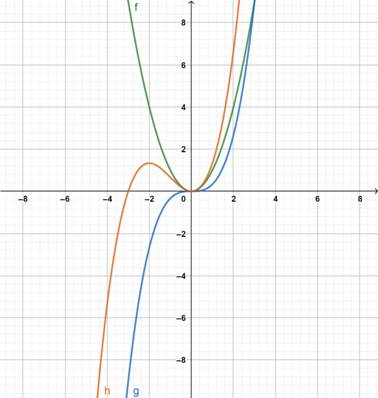# Draw the graph of f , g and f + g on a common graph### Precalculus: Mathematics for Calcu...

6th Edition
Stewart + 5 others
Publisher: Cengage Learning
ISBN: 9780840068071### Precalculus: Mathematics for Calcu...

6th Edition
Stewart + 5 others
Publisher: Cengage Learning
ISBN: 9780840068071

#### Solutions

Chapter 2.6, Problem 19E
To determine

## Draw the graph of f,g and f+g on a common graph

Expert Solution

### Explanation of Solution

Given information : the function is

f(x)=x2,g(x)=13x3

Graph : Sketch the graph using graphing utility.

Step 1: Press WINDOW button to access the Window editor.

Step 2: Press Y= button.

Step 3: Enter the expression f(x)=x2,g(x)=13x3 which is required to graph.

Step 4: Press GRAPH button to graph the function.

The graph is obtained as:Interpretation : On graph the green line shows the f(x)

And the blue line shows the g(x)

And the brown line shows (f+g)(x)

### Have a homework question?

Subscribe to bartleby learn! Ask subject matter experts 30 homework questions each month. Plus, you’ll have access to millions of step-by-step textbook answers!